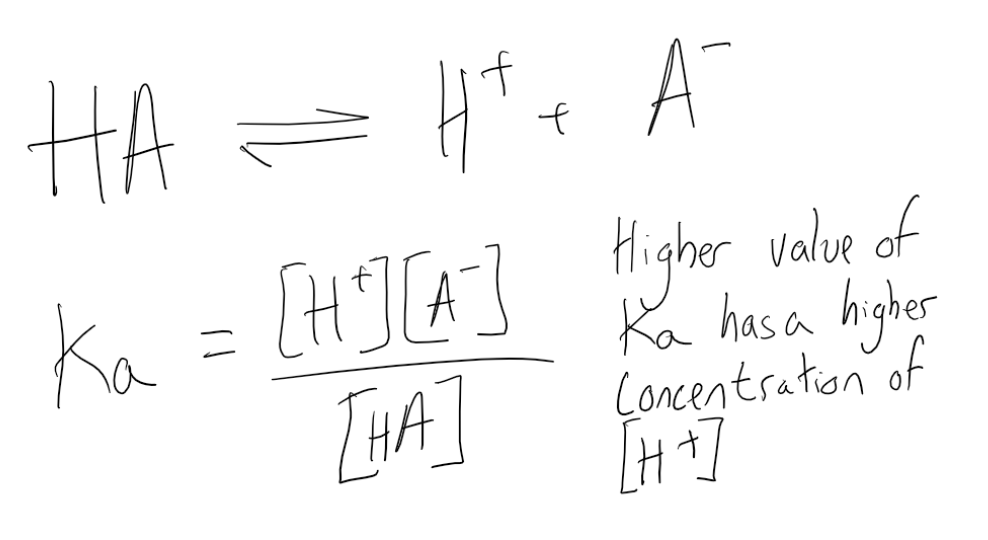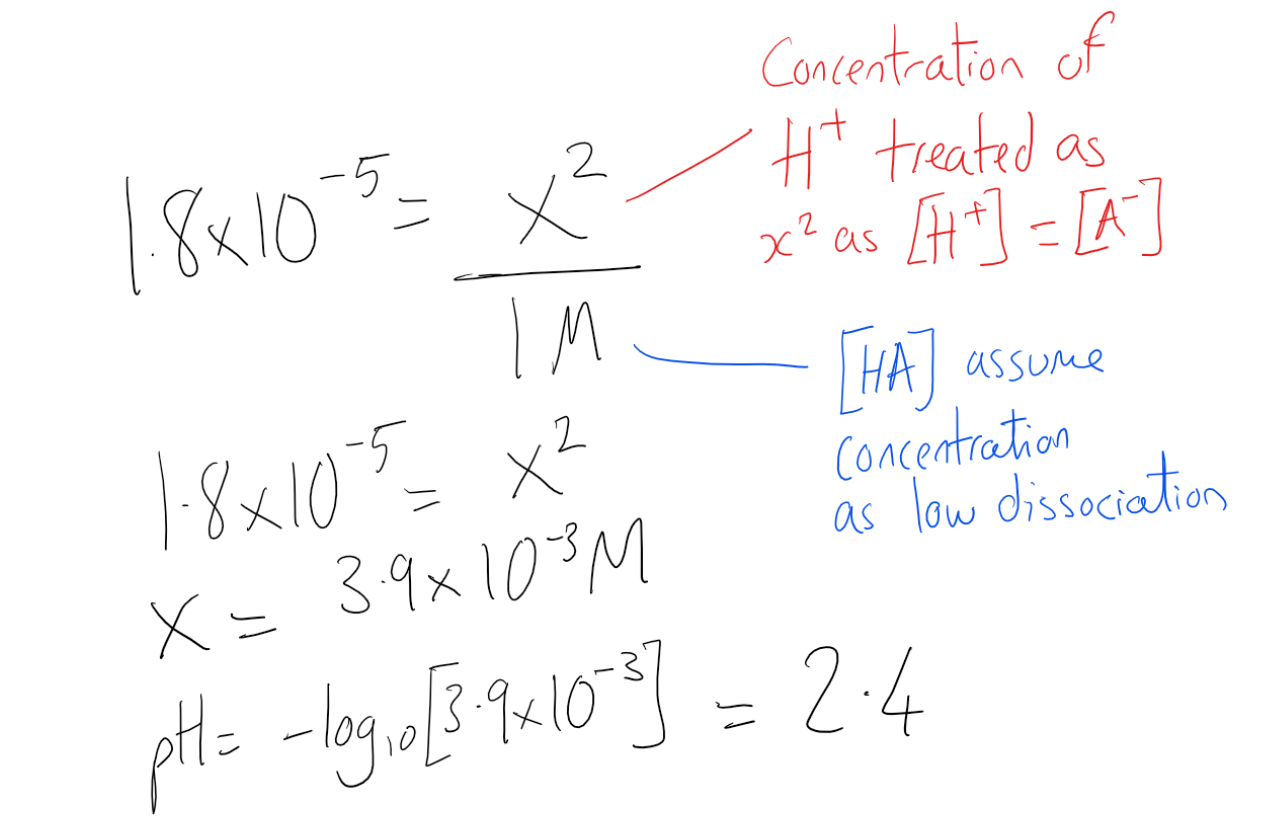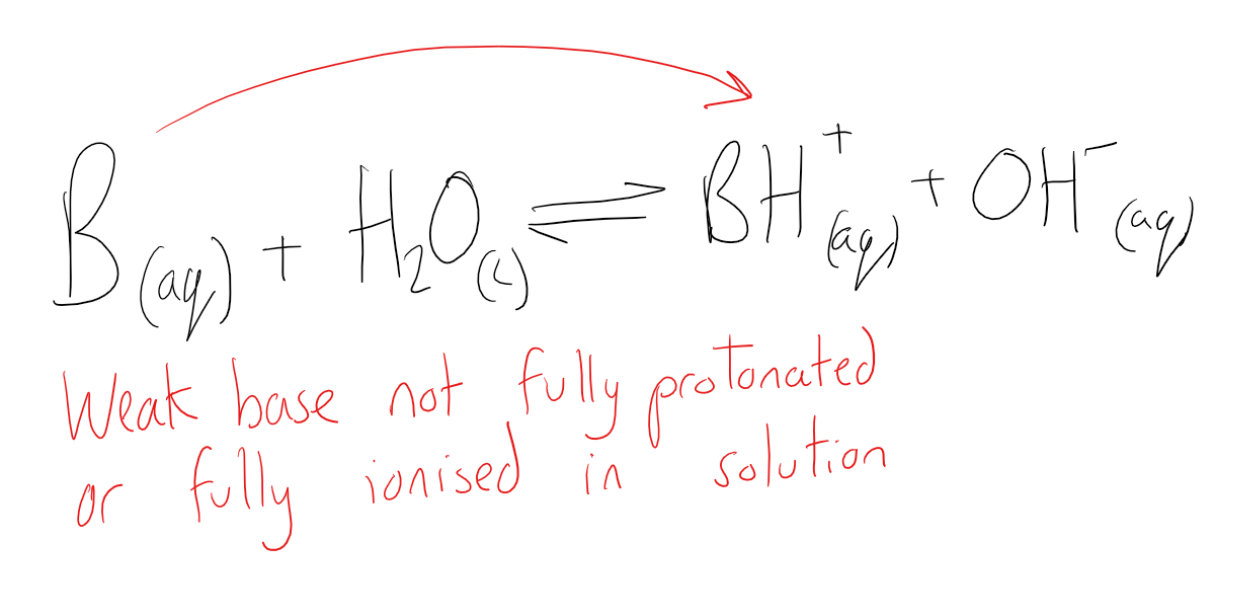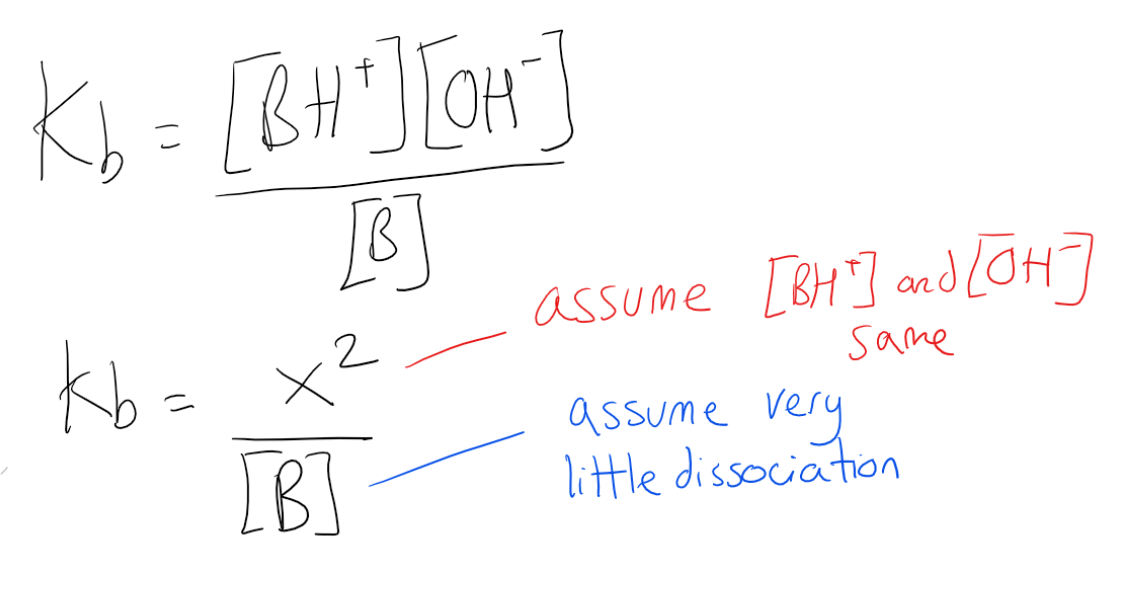Complimentary 1-hour tutoring consultation

MCAT Content / Acid Base Equilibria / Weak Acids And Bases

### Weak Acids and Bases

Topic: Acid Base Equilibria

A weak acid is one that does not dissociate completely in solution while a weak base is a chemical base that does not ionize fully in an aqueous solution.

Weak Acids
A weak acid is one that does not dissociate completely in solution; this means that a weak acid does not donate all of its hydrogen ions (H+) in a solution. Weak acids have very small values for Ka (and therefore higher values for pKa) compared to strong acids, which have very large Ka values (and slightly negative pKa values).

The majority of acids are weak. On average, only about 1 percent of a weak acid solution dissociates in water in a 0.1 mol/L solution. Therefore, the concentration of H+ ions in a weak acid solution is always less than the concentration of the undissociated species, HA. Examples of weak acids include acetic acid (CH3COOH), which is found in vinegar, and oxalic acid (H2C2O4), which is found in some vegetables.

Weak acids ionize in a water solution only to a very moderate extent. The generalized dissociation reaction is given below, where HA is the undissociated species and A is the conjugate base of the acid. The strength of a weak acid is represented as either an equilibrium constant or a percent dissociation. The equilibrium concentrations of reactants and products are related by the acid dissociation constant expression, Ka.The greater the value of Ka, the more favored the H+ formation, which makes the solution more acidic; therefore, a high Ka value indicates a lower pH for a solution. The Ka of weak acids varies between 1.8×10−16 and 55.5. Acids with a Ka less than 1.8×10−16 are weaker acids than water. If acids are polyprotic, each proton will have a unique Ka.

The pH of a weak acid can be found using the Ka value. But key assumptions need to be made. Firstly that the concentration of the dissociated acid and conjugate base H+ and A are equal. Also that the concentration of HA is the same as the given concentration due to such low levels of dissociation. Using the Ka equation we can then rearrange to isolate the concentration of H+ ions and put into the equation for pH.Weak Bases
A base is a substance that can accept hydrogen ions (H+) or, more generally, donate a pair of valence electrons. A weak base is a chemical base that does not ionize fully in an aqueous solution. As Brønsted-Lowry bases are proton acceptors, a weak base may also be defined as a chemical base with incomplete protonation. A general formula for base behavior is as follows:A base can either accept protons from water molecules or donate hydroxide ions to a solution. Both actions raise the pH of the solution by decreasing the concentration of H+ ions. This results in a relatively low pH compared to that of strong bases. The pH of bases in aqueous solution ranges from greater than 7 (the pH of pure water) to 14 (though some bases have pH values greater than 14). The formula for pH is:

pH = -log10[H+]

Sometimes, however, it is more convenient to focus on the pOH of bases, rather than the pH. The pOH more directly references the [OH].

pOH = -log10[OH]

To find the pH of a weak base depends on the strength of the base (given by Kb) and the concentration of the base (the molarity, or moles of the base per liter of solution):Some common weak bases and their corresponding pKb values include:

• C6H5NH2 (9.38)
• NH3 (4.75)
• CH3NH2 (3.36)
• CH3CH2NH2 (3.27)

Smaller pKb values indicate higher values of Kb; this also indicates a stronger base.

Like weak acids, weak bases have important applications in biochemical studies, chemistry reactions, and physiological purposes, particularly because of their role in buffer solutions. Weak bases can also be used to catalyze certain reactions.

Practice Questions

MCAT Official Prep (AAMC)

Official Guide C/P Section Passage 5 Question 21

Chemistry Question Pack Passage 9 Question 50

Sample Test C/P Section Passage 3 Question 11

Key Points

• The dissociation of weak acids, which are the most popular type of acid, can be calculated mathematically and applied in experimental work.

• If the concentration and Ka of a weak acid are known, the pH of the entire solution can be calculated. The exact method of calculation varies according to what assumptions and simplifications can be made.

• Weak acids and weak bases are essential for preparing buffer solutions, which have important experimental uses.

• A base is a substance that can accept hydrogen ions (H+) or, more generally, donate a pair of valence electrons; a weak base does not, therefore, fully ionize or completely accept hydrogen ions in an aqueous solution.

• Bases increase pH; weak bases have a less dramatic effect on pH.

• pOH is occasionally used as an alternative to pH to quantify the relative H+/hydroxide concentration in solution.

• A base dissociation constant, Kb, mathematically represents the base’s relative strength and is analogous to the acid dissociation constant; weaker bases have smaller Kb values.

• Like weak acids, weak bases can be used to make buffer solutions.

Key Terms

weak acid: One that dissociates incompletely, donating only some of its hydrogen ions into solution

Conjugate base: The species created after donating a proton.

Conjugate acid: The species created when a base accepts a proton

Enolate: A deprotonated enol

Enol: An organic alcohol with an -OH functional group located off a double bond

Weak base: A proton acceptor that does not ionize fully in an aqueous solution.

Billing Information